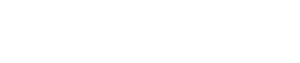Welcome to the renewed Beamex.com – to give feedback click here >

# Confidence interval

Beamex / Dictionary / Calibration uncertainty / Confidence interval

A range of values that is expected to contain the true value of the parameter being evaluated with a specified level of confidence. The confidence interval is calculated from sample statistics. Confidence intervals can be calculated for points, lines, slopes, standard deviations, and so on. For an infinite (or very large compared to the sample) population, the confidence interval is:where
CI is the confidence interval,
n is the number of items in the sample,
p is the proportion of items of a given type in the population,
s is the sample standard deviation,
x is the sample mean, and
t is the Student’s T value for α⁄2 and (n – 1) (α is the level of
significance).

Calibration uncertainty